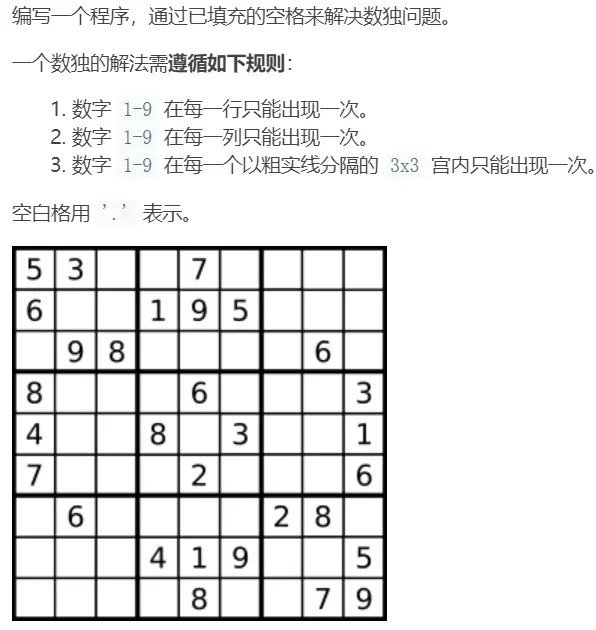# leetcode 37. Solve Sudoku backtracking template

Posted May 27, 20202 min readBefore solving this problem, you need to understand what is the backtracking method.

Generally speaking, the backtracking method is:there are currently multiple choices, we don't know which one to choose, we need to try each one. Each attempted path is connected to get a tree solution.

For example, the position of [[0,2]`in the coordinates of the above figure. Through observation, we can know that the numbers that can be placed at this position are:` 1, 2, 4`. If we choose to place `1` at our current position The numbers that can be placed at the next position are:`2, 6`. If we choose to place` 2` at our current position, the numbers at the next position can be placed:`1, 6`. Through analysis, a tree-shaped solution can be drawn:/**
* @param {character [][]} board
* @return {void} Do not return anything, modify board in-place instead.
* /
var solveSudoku = function(board) {
//auxiliary function to determine whether it is feasible to place a value
function isOk(i, j, val) {
//Determine whether the number already exists in the current row
for(let k = 0; k <9; k ++) {
if(board [i][k]== val) return false
}
//Determine if the number already exists in the current column
for(let k = 0; k <9; k ++) {
if(board [k][j]== val) return false
}
//Determine whether the number already exists in the current 3 * 3 grid
let l = Math.floor(j/3) * 3, t = Math.floor(i/3) * 3
for(let m = t; m <t + 3; m ++) {
for(let n = l; n <l + 3; n ++) {
if(board [m][n]== val) return false
}
}
return true
}
function backTrace(i, j) {
if(j === 9) {
//Sudoku is finished, return true
if(i === 8) return true
//otherwise go to the next line
i ++
j = 0
}
//There is no number in the current position
if(board [i][j]=== '.') {
//Choose the number from 1-9 and fill in
for(let val = 1; val <= 9; val ++) {
//If the current number meets the conditions, write to Sudoku
if(isOk(i, j, val)) {
board [i][j]= val + ''
//If there is a solution on this path, return directly if the condition is met
if(backTrace(i, j + 1)) return true
}
board [i][j]= '.'
}
} else {
//There is a number at the current position, go directly to the next box
return backTrace(i, j + 1)
}
//If the current cell has neither a number nor a number that satisfies the condition, it can be filled in,
//Explain that this path does not work
return false
}
backTrace(0,0)
};